ECE 476 Final Project: LED Sensor Piano Keyboard

Cristina Guzman (cgg27) and Joe Vulih (jpv23)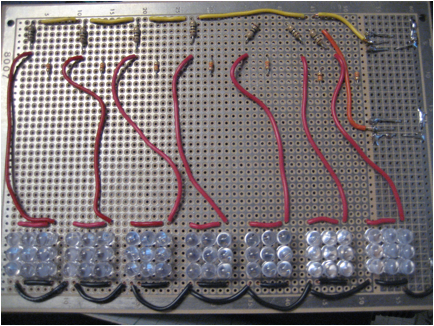# Contents

Introduction

·

·

· Logical Structure

· Standards

· Patents

Program Design

Hardware Design

·

·

· Safety

· Interference

· Usability

·

· Applicable standards

·

·

·

Appendix A: Commented Code

Appendix E: References

# Introduction

Our project utilizes an array of LEDs that work as light sensors to generate a musical tone, simulating a piano keyboard.

The basic idea is to use LEDs as both emitters and sensors. For our project specifically, we used a total of 63 LEDs, 9 for each of the seven natural keys. Only the middle LED served as photodetector when the key was pressed, absorbing light from the other LEDs reflected from the finger. We then used the Karplus-Strong algorithm for sound generation, all programmed in C.  The tone was passed through an audio amplifier, and can be listened through headphones or speakers connected to the audio jack.

We chose this project because we wanted to do something with LEDs as sensors, and specifically chose music because of our interest in this matter.

# High level design

·

·

· Logical Structure

· Standards

· Patents

### Rationale

The rationale behind this idea consists of using a diode in a 3 step process, as shown in the following schematic. Each step is approximately 1 millisecond long.  The upper pin will starts at Vcc and stay there for one millisecond (step one), and then drop to ground for two milliseconds (step two and three). The lower pin will start at ground for step 1, then rise to Vcc for the length of step two and finally the diode will discharge exponentially due to the hole-electron generation due to the incident light on the LED (8 pin leakages).For the sound generation we will use the Karplus-Strong algorithm developed in the 80s, which is computationally simple. It is a method of physical modeling a string that simulates the sound of some types of instruments, including the piano. It uses a low pass filter and a delay block, which we will implement in software. A schematic of how it works is shown below.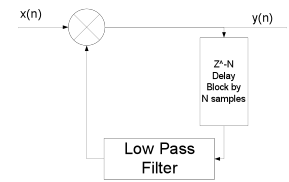### Background Math

The output frequency for each note was calculated from the formula,Where N is the length of the delay line, and fs is the sample frequency at which the loop is updated.

For this project we used a sample rate of 8 kHz. While this sample rate causes some quantization of possible frequencies since the delay line has only integer lengths, we were still able to get relatively accurate frequency synthesis.

The input x(n) is excited using a signal noise until the delay line is filled to excite the entire system.

The low pass filter is simply a moving average, which has the equation below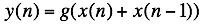where g is a loss factor that must be less than 0.5 for the system to be stable. By making g smaller the signal will die out faster.

### Logical structure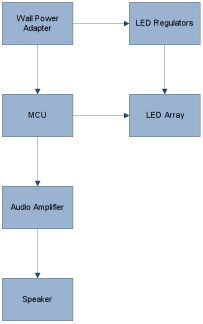Above is a block diagram of the system wiring. The MCU interfaces with the LED array to get the human touch interfaces, and then produces a sound output that is passed through the audio amplifier to the speakers.

We implemented a low pass filter in software for the Karplus-Strong algorithm. Even though low pass filters can be made with hardware, for this part it was necessary to do it in software.

Also, while we implemented the Karplus-Strong in software, the algorithm would work much faster in hardware, but we did not have the expertise nor the time to design on the algorithm on an FPGA.

We also decided to use integers for our variables in our code instead of floats because even though this gives less accuracy, it is faster and timing was more of an issue.

### Standards

One standard that we followed in our design is ISO 16:1975 that specifies that in music the A above middle C is 440 Hz.

### Patents

Some intellectual property rights we considered are two patents, both concerning the Karplus-Strong algorithm. One patent is the “Wavetable-modification instrument and method for generating musical sound” (Patent Number 4,649,783) and the other is “Independently controlled wavetable-modification instrument and method for generating musical sound” (4,622,877). They are both expired, since they were filed in the early 80s.

# Program design

The software was grouped into two functions. The first was the sensing of the LEDs and the second was the generation of the audio tones.

The LED sensing was controlled by a state machine that updated approximately every millisecond and scanned the entire keypad one button at a time. Since we only had 7 buttons, we were able to scan the keypad in around 16 ms. This routine would then either reinitialize the Karplus-Strong routine for a specific button, or it would change the damping on the string to shut off a button.

The Karplus-Strong sound synthesis was always running. The strings were always being updated, and whether they were playing audibly or not was depended on the damping on the string. The button routine would set the damping really high if the button was not pressed. This is equivalent to the strings of the piano always being present, except being heavily damped.

A part that was tricky to write the part of summing of each of the outputs from the Karplus-Strong. This was difficult due to the fact that we were using only a 10 bit PWM on timer 1. To do this we first added up all the outputs as longs, then, since they are represented using 16 bits, we right shifted the value by 9 bits since the addition of 7 16 bit numbers could produce 19 bits of output. Then an offset was added to center the PWM at the middle of its range.

# Hardware design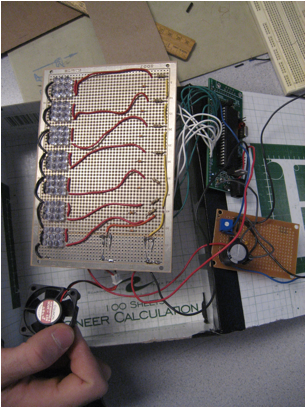The major hardware components were the 63 Ultra Red 1000mcd 5mm LEDs. These 1-inch LEDs have a water clear epoxy. The maximum forward current is 20mA, with a maximum forward drop of 2.0 V and maximum reverse voltage of 5 V. All these were soldered to the large bread board, by arranging them by 9 LEDs (3 rows, 3 columns) per key. To limit the current through the LEDs a 10 ohm resistor was used for the 8 non sensor LEDs in each key, and a 330 ohm resistor was used for the sensor LED. The rims of the LEDs were trimmed using the Dremel tool to remove the flange to get the LEDs to fit closer together.

One thing that we tried and did not work was to use the oscilloscope while testing. This was because the scope loaded the LEDs too much and corrupted their discharge time, so the device would not operate properly.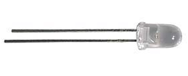Two LM317TFS Voltage Regulators were used, which are capable of supplying 1.5 A of current, with an adjustable output voltage from 1.2V to 37V. They were configured to output approximately 3.2 V for the LEDs.

One regulator was used for 4 keys, while the other was used for the other 3. A small piece of aluminum sheeting was used to make a heat sink for each of them, and a fan was used to keep these regulators cool, as seen in the picture below.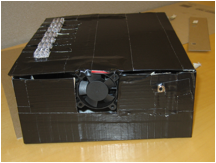For the output we used an LM386 audio power amplifier with a variable gain from 20 to 200. Set up the device to use a gain of 20 to prevent saturating the output. It came in an 8 pin DIP package, which can be seen in the picture below.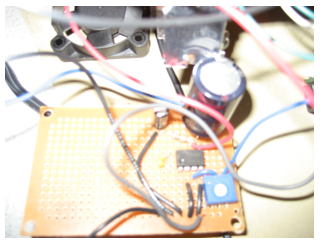The Atmel ATMega 664 was used to control the entire system. This was mounted on a board that includes a power supply, crystal clock, and programming interface. A six-pin header allows flash memory programming from an STK500 or other Atmel programmer.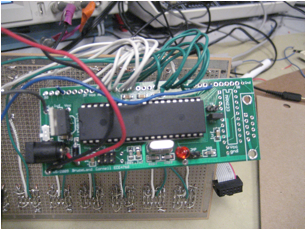# Results of the design

·

·

· Safety

· Interference

· Usability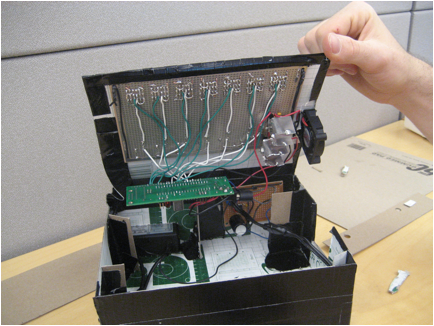### Speed of Execution

Timer 0, controlled of the polling of the LEDs, was ran at a speed of approximately 1millisecond. This updated the state machine, which had 16 total states. This meant that we could scan the entire LED array is approximately 16 ms. The actual polling of the LEDs was put in another routine so that it could be interrupted by the much faster Timer 1 ISR.

Timer 1 was executed at a speed of 8kHz to control the sampling of the Karplus-Strong algorithm.

Timer 2 was clocked at 20 MHz to generate the PWM output for the audio signal on pin D5.

### Accuracy

The frequencies for the 7 naturals used in our keyboard are:

 Note Frequency (Hz) Wavelength (cm) C4 261.63 132 D4 293.66 117 E4 329.63 105 F4 349.23 98.8 G4 392.00 88.0 A4 440.00 78.4 B4 493.88 69.9

### Safety

There are no safety considerations with our project since we are not using extremely bright LEDs (which could affect the retina) nor will the volume be too high to affect the human ear. However, as with any light source, like a bulb, staring at it for a prolonged period of time could damage the eyes.

### Interference

Our project does not radiate any harmful signals that would interfere with any electric circuits to our knowledge. And our project accepts all interference.

### Usability

Our product will be used as recreation for people interested in music and playing the piano. People with special needs will not be using our product, with the exception of the blind. They could play the notes but will not be able to appreciate the LEDs. The behavior of the LED buttons varies based on skin reflectivity; however, we have calibrated the sensitivity to respond to the lowest reflectivity. However, this means the interface will behave differently with skin tones.

# Conclusions

·

· Applicable standards

·

·

·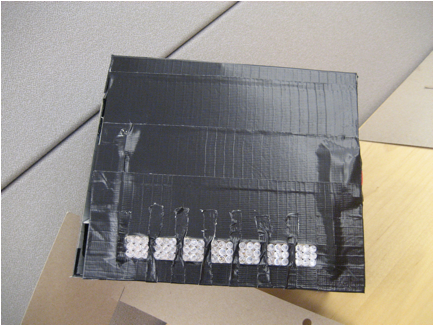### Expectations

In conclusion, our final project met all the specs that we initially set out to do. We used LEDs as sensors to simulate a piano octave. We used the Karplus-Strong algorithm to accurately generate sound. We stayed well below the \$75 dollar limit, and even spent less than we predicted.

While we are happy with out results, there are some things that could be improved. First of all, we could increase the accuracy of the PWM from 12 bit to 16 bit. We could also add the flat/sharp tones to the octave, as a piano has. Finally we could use a plastic enclosure instead of a cardboard one.

### Applicable standards

The only standard we had to follow has that A440 is the standard for musical pitch, the ISO 16:1975. As it is seen in the accuracy results section, we did follow this standard for all notes in our octave, beginning with middle C.

### Intellectual property considerations

There were no intellectual property considerations since we did not reuse someone’s code or design. We did not use code from the public domain either. Or reverse engineering. Although we used the Karplus-Strong algorithm, this patent expired so there were no issues.

We also did not sample any parts that required non-disclosure forms to be signed.

There are no current patent or publishing opportunities for this project.

### Ethical considerations

Since we are committed to the highest ethical and professional conduct, we abided by the IEEE Code of Ethics when developing this project. We were consistent specifically with rules 7, 8 and 10. Rule 7 states that we agree “to seek, accept, and offer honest criticism of technical work, to acknowledge and correct errors, and to credit properly the contributions of others”. We credited the Karplus-Strong Algorithm for sound generation, as well as sought and accepted help from our professor Bruce Land and other teaching assistants. We also credit our several sources that gave us information about using LEDs as sensors.

Rule 8 states “to treat fairly all persons regardless of such factors as race, religion, gender, disability, age, or national origin”. We took this into consideration when setting our detection threshold, since different skin tones respond in distinct ways. Our LED piano senses even the least reflective skin tones, so there is no discrimination towards race. Also any person, from any country, of any age and gender can use our project. As we stated before, people with disabilities will not be using our product, with the exception of the blind.

Rule 10 affirms that we are to “to assist colleagues and co-workers in their professional development and to support them in following this code of ethics”.  There is another group that is also producing musical strings for their project, and when they asked for help we supported them by giving them advice on what we had done. We also offered advice to other groups working with LED and getting them to fit on the bread boards which had pin spacings that were rather small.

### Legal considerations

There are no legal considerations, since all patents have expired.

# Appendix A: Commented Code

#include <inttypes.h>

#include <avr/io.h>

#include <avr/interrupt.h>

#include <avr/sleep.h>

#include <util/delay.h>

#include <math.h>

#include <stdlib.h>

#define t1 1 // 19 for timer 1 ovf

#define begin {

#define end }

#define g_on 0.49999

#define g_off 0.001

//=== fixed conversion macros =========================================

#define int2fix(a)  (((int)(a))<<8)         //Convert char to fix. a is a char

#define fix2int(a)   ((uint8_t)((a)>>8))    //Convert fix to int. a is an int

#define float2fix(a) ((int)((a)*256.0))         //Convert float to fix. a is a float

#define fix2float(a) (((float)(a))/256.0)       //Convert fix to float. a is an int

// identify the assembler routine to C

extern int multfix(int a,int b);

void pollLED(void);

void initialize(void); //all the usual mcu stuff

volatile unsigned char time1 ;      //timeout counters

//Global Variables

unsigned char var;

unsigned char keyNum;

signed int init_str;

volatile signed int g_off_fix;

volatile signed int g_on_fix;

// C4 Variables

volatile signed int APF_C4;

volatile signed int LPF_C4;

volatile int N_C4=59;

volatile signed int delay_C4;

volatile char out_C4;

volatile char last_C4;

volatile char play_C4;

volatile int C4_ocr;

volatile signed int g_C4;

// D4 Variables

volatile signed int APF_D4;

volatile signed int LPF_D4;

volatile int N_D4=53;

volatile signed int delay_D4;

volatile char out_D4;

volatile char last_D4;

volatile char play_D4;

volatile int D4_ocr;

volatile signed int g_D4;

// E4 Variables

volatile signed int APF_E4;

volatile signed int LPF_E4;

volatile int N_E4=47;

volatile signed int delay_E4;

volatile char out_E4;

volatile char last_E4;

volatile char play_E4;

volatile int E4_ocr;

volatile signed int g_E4;

// F4 Variables

volatile signed int APF_F4;

volatile signed int LPF_F4;

volatile int N_F4=44;

volatile signed int delay_F4;

volatile char out_F4;

volatile char last_F4;

volatile char play_F4;

volatile int F4_ocr;

volatile signed int g_F4;

// G4 Variables

volatile signed int APF_G4;

volatile signed int LPF_G4;

volatile int N_G4=39;

volatile signed int delay_G4;

volatile char out_G4;

volatile char last_G4;

volatile char play_G4;

volatile int G4_ocr;

volatile signed int g_G4;

// A440 Variables

volatile signed int APF_A440;

volatile signed int LPF_A440;

volatile int N_A440=35;

volatile signed int delay_A440;

volatile char out_A440;

volatile char last_A440;

volatile char play_A440;

volatile int A4_ocr;

volatile signed int g_A4;

// B4 Variables

volatile signed int APF_B4;

volatile signed int LPF_B4;

volatile int N_B4=31;

volatile signed int delay_B4;

volatile char out_B4;

volatile char last_B4;

volatile char play_B4;

volatile int B4_ocr;

volatile signed int g_B4;

volatile signed int temp;

char forward;

//**********************************************************

//timer 2 compare ISR which controls the timing of the LEDs

ISR (TIMER2_COMPA_vect)

begin

--time1;

end

// Audio Output ISR

ISR (TIMER0_COMPA_vect) {

//PORTB=PORTB ^ 0x08;

if(play_C4 == 1) {

// update output OCR

C4_ocr = (delay_C4[out_C4]);

delay_C4[last_C4]= multfix(g_C4,delay_C4[last_C4]+C4_ocr);

// Check for pointer wrap around

if(out_C4==(N_C4)){

out_C4=0;

}else {

out_C4++;

}

if(last_C4==(N_C4)){

last_C4=0;

}else {

last_C4++;

}

}

if(play_D4 == 1) {

D4_ocr = (delay_D4[out_D4]);

delay_D4[last_D4]= multfix(g_D4,delay_D4[last_D4]+D4_ocr);

if(out_D4==(N_D4)){

out_D4=0;

}else {

out_D4++;

}

if(last_D4==(N_D4)){

last_D4=0;

}else {

last_D4++;

}

}

if(play_E4 == 1) {

E4_ocr = (delay_E4[out_E4]);

delay_E4[last_E4]= multfix(g_E4,delay_E4[last_E4]+E4_ocr);

if(out_E4==(N_E4)){

out_E4=0;

}else {

out_E4++;

}

if(last_E4==(N_E4)){

last_E4=0;

}else {

last_E4++;

}

}

if(play_F4 == 1) {

F4_ocr = (delay_F4[out_F4]);

delay_F4[last_F4]= multfix(g_F4,delay_F4[last_F4]+F4_ocr);

if(out_F4==(N_F4)){

out_F4=0;

}else {

out_F4++;

}

if(last_F4==(N_F4)){

last_F4=0;

}else {

last_F4++;

}

}

if(play_G4 == 1) {

G4_ocr = (delay_G4[out_G4]);

delay_G4[last_G4]= multfix(g_G4,delay_G4[last_G4]+G4_ocr);

if(out_G4==(N_G4)){

out_G4=0;

}else {

out_G4++;

}

if(last_G4==(N_G4)){

last_G4=0;

}else {

last_G4++;

}

}

if(play_A440 == 1) {

A4_ocr=(delay_A440[out_A440]);

delay_A440[last_A440]= multfix(g_A4,delay_A440[last_A440]+A4_ocr);

if(out_A440==(N_A440)){

out_A440=0;

}else {

out_A440++;

}

if(last_A440==(N_A440)){

last_A440=0;

}else {

last_A440++;

}

}

if(play_B4 == 1) {

B4_ocr = (delay_B4[out_B4]);

delay_B4[last_B4]= multfix(g_B4,delay_B4[last_B4]+B4_ocr);

if(out_B4==(N_B4)){

out_B4=0;

}else {

out_B4++;

}

if(last_B4==(N_B4)){

last_B4=0;

}else {

last_B4++;

}

}

//Sum the OCR variables of all the keys, and perform a right shift to prevent overflow, then add an offset

OCR1A = ((((long)C4_ocr + (long)D4_ocr + (long)E4_ocr + (long)F4_ocr + (long)G4_ocr + (long)A4_ocr + (long)B4_ocr))>>9) + 512;

}

//**********************************************************

//Entry point and task scheduler loop

int main(void)

begin

initialize();

DDRC = 0xff;

PORTC=0xff;

while(1)

begin

// reset time and call task

if (time1==0){ time1=t1; pollLED();}

end

end

//*******************************

//Polls the LED sensors to see if the current one being read is on

//and provides visual output recognition

void pollLED(void) {

if (var==0){

//forward bias all

DDRA = 0xff;

PORTA = 0x00;

PORTC = 0xff;

var = 1;

} else if (var==1) {

//reverse bias key 1, forward bias the rest

DDRA  = 0b11111111;

PORTA = 0b00000001;

PORTC = 0b11111110;

var=2;

} else if (var==2) {

// Key 2, PinA high output, Pin C low output

// Key 1, PinA low input, Pin C low output

// forward bias the rest

DDRA  = 0b11111110;

PORTA = 0b00000010;

PORTC = 0b11111100;

// Set mux to key 1

var=3;

} else if (var==3) {

var = 4;

} else if (var==4) {

// ADC value has finished converting, determine press

// Determine if the key was pressed

// If so, was it pressed the last time it was polled

if(g_C4 == g_off_fix) {

// if not, then load the delay line and set the damping to that of a pressed key

play_C4 = 0;

for(int i=0; i<=N_C4;i++){

delay_C4[i]= init_str[i];

}

g_C4 = g_on_fix;

play_C4 = 1;

}

} else {

// Other wise set the damping to an off key

g_C4 = g_off_fix;

}

//Key 3, Pin A high output, Pin C low output

//Key 2, Pin A low input, PinC low output

DDRA  = 0b11111101;

PORTA = 0b00000100;

PORTC = 0b11111001;

// Set mux to key 1

var=5;

} else if (var==5) {

var = 6;

} else if (var==6) {

// ADC value has finished converting, determine press

if(g_D4 == g_off_fix) {

play_D4 = 0;

for(int i=0; i<=N_D4;i++){

delay_D4[i]= init_str[i];

}

g_D4 = g_on_fix;

play_D4 = 1;

}

} else {

g_D4 = g_off_fix;

}

//Key 4, Pin A high output, Pin C low output

//Key 3, Pin A low input, PinC low output

DDRA  = 0b11111011;

PORTA = 0b00001000;

PORTC = 0b11110011;

// Set mux to key 1

var=7;

} else if (var==7) {

var = 8;

} else if (var==8) {

// ADC value has finished converting, determine press

if(g_E4 == g_off_fix) {

play_E4 = 0;

for(int i=0; i<=N_E4;i++){

delay_E4[i]= init_str[i];

}

g_E4 = g_on_fix;

play_E4 = 1;

}

} else {

g_E4 = g_off_fix;

}

//Key 5, Pin A high output, Pin C low output

//Key 4, Pin A low input, PinC low output

DDRA  = 0b11110111;

PORTA = 0b00010000;

PORTC = 0b11100111;

// Set mux to key 1

var=9;

} else if (var==9) {

var = 10;

} else if (var==10) {

// ADC value has finished converting, determine press

if(g_F4 == g_off_fix) {

play_F4 = 0;

for(int i=0; i<=N_F4;i++){

delay_F4[i]= init_str[i];

}

g_F4 = g_on_fix;

play_F4 = 1;

}

} else {

g_F4 = g_off_fix;

}

//Key 6, Pin A high output, Pin C low output

//Key 5, Pin A low input, PinC low output

DDRA  = 0b11101111;

PORTA = 0b00100000;

PORTC = 0b11001111;

// Set mux to key 1

var=11;

} else if (var==11) {

var = 12;

} else if (var==12) {

// ADC value has finished converting, determine press

if(g_G4 == g_off_fix) {

play_G4 = 0;

for(int i=0; i<=N_G4;i++){

delay_G4[i]= init_str[i];

}

g_G4 = g_on_fix;

play_G4 = 1;

}

} else {

g_G4 = g_off_fix;

}

//Key 7, Pin A high output, Pin C low output

//Key 6, Pin A low input, PinC low output

DDRA  = 0b11011111;

PORTA = 0b01000000;

PORTC = 0b10011111;

// Set mux to key 1

var=13;

} else if (var==13) {

var = 14;

} else if (var==14) {

// ADC value has finished converting, determine press

if(g_A4 == g_off_fix) {

play_A440 = 0;

for(int i=0; i<=N_A440;i++){

delay_A440[i]= init_str[i];

}

g_A4 = g_on_fix;

play_A440 = 1;

}

} else {

g_A4 = g_off_fix;

}

//Key 7, Pin A low input, PinC low output

DDRA  = 0b10111111;

PORTA = 0b00000000;

PORTC = 0b10111111;

// Set mux to key 1

var=15;

} else if (var==15) {

var = 16;

} else if (var==16) {

// ADC value has finished converting, determine press

if(g_B4 == g_off_fix) {

play_B4 = 0;

for(int i=0; i<=N_B4;i++){

delay_B4[i]= init_str[i];

}

g_B4 = g_on_fix;

play_B4 = 1;

}

} else {

g_B4 = g_off_fix;

}

//Key 5, Pin A high output, Pin C low output

//Key 4, Pin A low input, PinC low output

DDRA  = 0b11111111;

PORTA = 0b00000000;

PORTC = 0b11111111;

// Set mux to key 1

var=1;

}

}

//**********************************************************

//Set it all up

void initialize(void) {

//set up the ports

// PORT A is used as the CATHODE (ground) of the sensing LEDs,

// which will be charged and floated each time the LED is polled

DDRA=0x0f;    // PORT A is an output

PORTA=0;

// PORT C is used as the ANODE (vdd) of the LEDs

DDRC = 0xff;

time1=t1;

//set variable for key poll sweep

keyNum = 0;

//init the A to D converter

//channel zero/ left adj /INTERNAL Aref

//!!!DO NOT CONNECT Aref jumper!!!!

//enable ADC and set prescaler to 1/128*20MHz=156,250

//and clear interupt enable

//and start a conversion

// The initial condition on the delay lines preloaded into an array

for(int i=0; i<100;i++){

init_str[i] = rand();

}

// Initiallize all the playback variables

g_off_fix = float2fix(g_off);

g_on_fix = float2fix(g_on);

g_C4=g_off_fix;

g_D4=g_off_fix;

g_E4=g_off_fix;

g_F4=g_off_fix;

g_G4=g_off_fix;

g_A4=g_off_fix;

g_B4=g_off_fix;

out_C4=1;

last_C4=0;

out_D4=1;

last_D4=0;

out_E4=1;

last_E4=0;

out_F4=1;

last_F4=0;

out_G4=1;

last_G4=0;

out_A440=1;

last_A440=0;

out_B4=1;

last_B4=0;

// Audio output

DDRD = 0xff;

// Timer 2 for Polling LEDs

TIMSK2 = (1 << OCIE2A);    // Timer 2 Interrupt Enable

OCR2A = 200;

TCCR2B = 5;                      // divide by 128 prescalar

TCCR2A= (1<<WGM21);        // Turn on clear on compare match

/* Timer 0 for update rate*/

// timer 0 runs at full rate

TCCR0B = 3;

//turn on timer 0 overflow ISR

TIMSK0 = 2 ;

TCCR0A = (1<<WGM01) ; // divide by 64

OCR0A = 39;

/* Timer 1 for 10 bit PWM operation */

// No Prescaling, Fast PWM 10 Bit

TCCR1B = (1<<WGM12) | (1<<CS10);

// Fast PWM 10 bit

TCCR1A = (1<<COM1A1) | (1<<COM1A0) | (1<<WGM11) |(1<<WGM10);

//crank up the ISRs

sei();

}

;******************************************************************************

;*

;* FUNCTION

;*    muls16x16

;* DECRIPTION

;*    Signed multiply of two 16bits numbers with 16 bits result.

;* USAGE

;*    r25:r24 = r23:r22 * r25:r24

;******************************************************************************

;int multfix(int a,int b)

.global multfix

multfix:

;input parameters are in r23:r22(hi:lo) and r25:r24

;b aready in right place -- 2nd parameter is in r22:23

mov  r20,r24 ;load a -- first parameter is in r24:25

mov  r21,r25

muls r23, r21     ; (signed)ah * (signed)bh

mov   r25, r0         ;r18, r0"

mul   r22, r20         ; al * bl"

mov  r24, r1      ;movw r17:r16, r1:r0"

mulsu r23, r20    ; (signed)ah * bl

mulsu r21, r22    ; (signed)bh * al

clr  r1                 ; required by GCC

;return values are in 25:r24 (hi:lo)

Ret

# AppendixB: schematics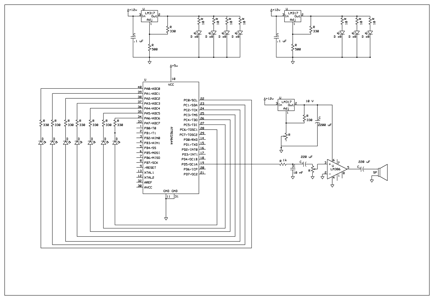# Appendix C: cost details

 Part Quantity Price LEDs 100 \$14 Resistors (309 ohms) 7 Free Resistors (10 Kohms) 7 Free Large bread board 1 Free (Surplus) Small bread board 1 \$1 ATMega664 1 \$8 Fan 1 \$1 Regulators (LM317TFS) 2 Free (Surplus) Protoboard 1 \$4 Audio Amplifier (LM386) 1 Free (Surplus) Total \$ 28

Here is a list of the specific tasks in the project carried out by each team member.

Writing the Code: Joe

Testing the Code: Both

Ordering and buying parts for the project: Joe

Designing the Hardware: Joe

Testing the Hardware: Both

Soldering the Parts: Both

Integration and final testing: Both

Writing the Report: Both

Creating the webpage: Cristina

# Appendix E: References

1. Data sheets

Audio Amplifier

ATMega644

2. Vendor sites

MPJA

3. Background sites/papers

Gordon McLellan's Blog, justDIY

Julius O. Smith III's Digital Waveguide Synthesis Page